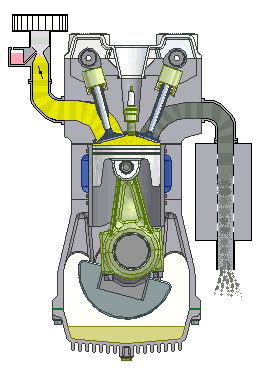# Volume flow rate of a piston

An engine's piston moves at an average speed of 10 $\text{m/s}$ while pulling the air-fuel mixture through a 3 $\text{cm}$ by 2 $\text{cm}$ rectangular intake valve. Find the average volume flow rate for the air-fuel mixture entering the piston in $\frac{\text{m}^3}{s}$. (Assume the piston has the same cross-sectional dimensions as the intake valve.)This four-stroke engine goes through the stages of intake, compression, combustion, and exhaust.

×